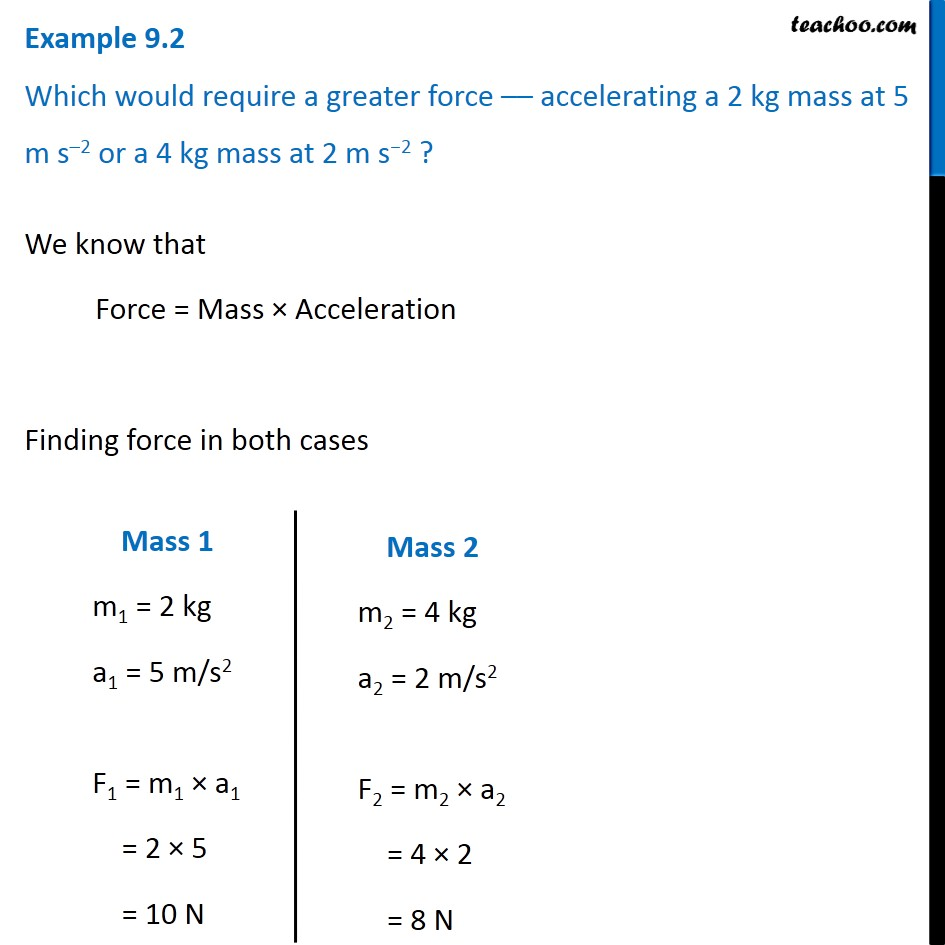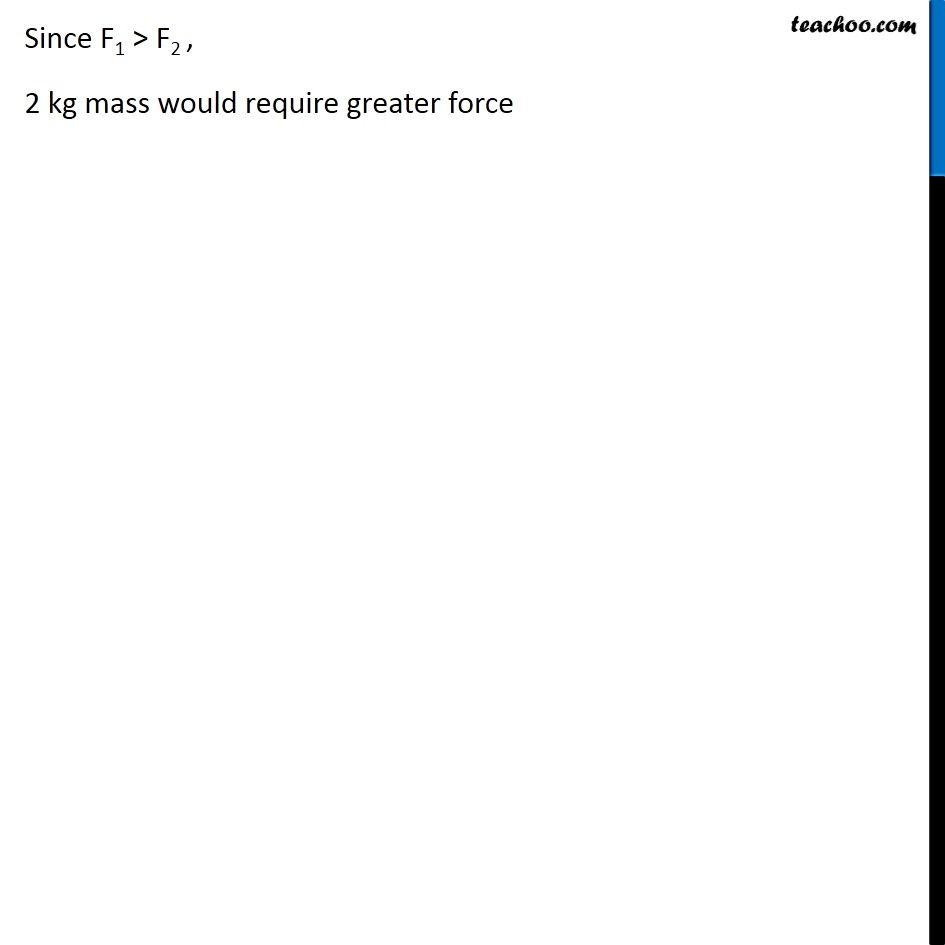Examples from NCERT Book

Class 9
Chapter 9 Class 9 - Force and Laws Of MotionLearn in your speed, with individual attention - Teachoo Maths 1-on-1 Class

### Transcript

Example 9.2 Which would require a greater force –– accelerating a 2 kg mass at 5 m s–2 or a 4 kg mass at 2 m s−2 ? We know that Force = Mass × Acceleration Finding force in both cases Mass 1 MAssm1 = 2 kg a1 = 5 m/s2 F1 = m1 × a1 = 2 × 5 = 10 N Mass 2 m2 = 4 kg a2 = 2 m/s2 F2 = m2 × a2 = 4 × 2 = 8 N Since F1 > F2 , 2 kg mass would require greater force